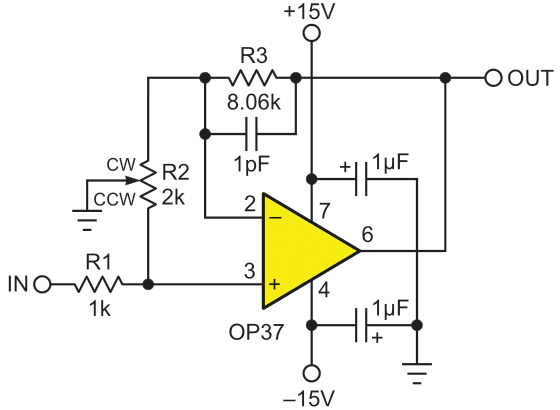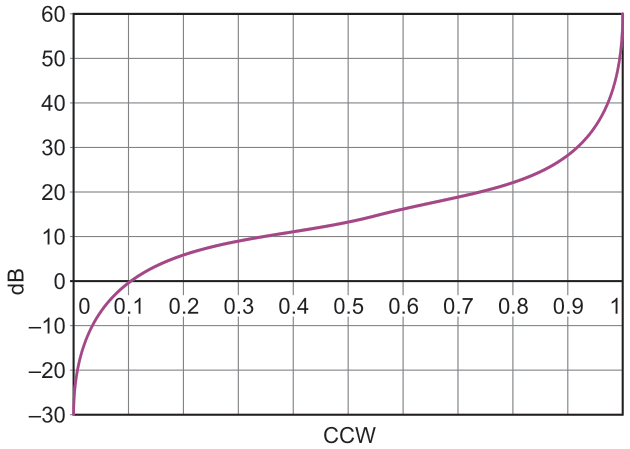# Adjust op-amp gain from -30 dB to +60 dB with one linear pot

## Analog Devices OP37

A good manually adjusted audio gain stage is characterized by:

• Low noise and low distortion,
• A 20 kHz bandwidth,
• A wide gain adjustment range (e.g. 90 dB), and
• Logarithmic (at least pseudo-logarithmic) control characteristic to
• Efficiently span that wide gain range with good resolution (not too twitchy)

Figure 1’s circuit checks all these boxes.Figure 1. Low noise DC to 20 kHz audio gain stage based on the OP37 with pseudo-logarithmic gain adjustment.

Figure 1 is based on the OP37 which has an impressive list of specs. They include a 60 MHz gain-bandwidth, making 20 kHz bandwidth compatible with gain up to 3000x = +70 dB, noise ~3 nV/√Hz which is less than the thermal noise of a 600 Ω resistor, ~20 µV input DC offset, etc.

Figure 1 exploits these impressive features while also accommodating one quirk. To ensure stability, the OP37 requires a minimum closed loop gain of 5 = 14 dB. Satisfying this requirement is assured by the R2:R3 ratio. Minimum closed loop gain = R3/R2 + 1 = 4.03 + 1 = 5.03.

But a minimum gain of 14 dB for the entire circuit would be incompatible with many audio applications, which often require a minimum adjustable gain < 1, or even approaching zero. This is the reason for the R1/R2 variable voltage divider, which sets a lower minimum-gain without incurring the cost of a dual potentiometer.

Grounding the pot wiper creates two mechanically linked but electrically independent variable resistors. The bottom half (ccw) cooperates with R1 to form a variable input attenuator with an (input) gain of:

GI = 2ccw/(1 + 2ccw)

ccw = fraction of R2 between ccw limit and wiper position.

So GI, the attenuator’s gain, goes from near zero to 2/3 as ccw goes from 0 to 1.

Meanwhile, the top half of the pot forms a variable feedback network with R2. This network sets the OP37’s closed loop (output) gain:

GO = (8.06/2cw + 1)

cw = fraction of R2 between wiper and cw limit

Cascading these two ratios (both controlled by the R2 pot), results in a total IN-to-OUT gain product of:

I/O gain = (2ccw/(1 + 2ccw))(8.06/2cw + 1)

Figure 2 shows the plot of the pseudo-logarithmic result with good log conformity from –10 dB to +40 dB.Figure 2. Pseudo-logarithmic GIGO gain versus R2 wiper position 0 to 1 = fully CCW to fully CW.

To optimize noise performance, R1 and R3 should be metal film types to avoid carbon-resistor excess (1/f) noise, and R2 should be a cermet type.

EDN